## TN State Board 12th Computer Science Important Questions Chapter 11 Database Concepts

Question 1.
Write the components of the Database management system?
There are five major components of the Data base management system. They are

1. Hardware
2. Software
3. Data
4. Methods
5. Database Access Language

Question 2.
What is an object model?

1. Object model is to store the data in the form of objects, attributes and methods, classes and Inheritance.
2. This model handles more complex applications, such as GIS, Engineering design.Question 3.
What is the roll of End user?

1. All modem computer Applications, web or mobile, store user data.
2. The End users are one who store, retrieve, update and delete data.

Question 4.
What is known as EF codd Rules?
Database normalization was first proposed by Dr. Edgar F Codd as an integral part of . RDBMS in order to reduce data redundancy and improve data integrity. These rules are known as EF codd mles.

Question 5.
What are unary Relational operations in Relational Algebra?
Unary Relational operations are

• SELECT (σ)
• PROJECT (Π)Question 6.
What are Relational Algebra operations from set theory?

• UNION (∪)
• INTERSECTION (∩)
• DIFFERENCE (-)
• CARTESIAN PRODUCT (×)

Question 7.
What is use of SELECT operation?
The SELECT operation used for selecting a subset with tuples according to a given condition.
The general form is σ<sub>c</sub> (R)
R – relation
C – attributes of R<sub>c</sub>

Question 8.
What is a PROJECT?

1. The projection eliminates all attributes of the input relation but those mentioned in the projection list.
2. The projection method defines a relation that contains a vertical subset of Relation.Question 9.
What are types of data model?
Types of data model are Hierarchical, Relational, Network, ER and object.

Question 10.
What is Data?
Data are raw facts stored in a computer. A data may contain any character, text, word or a number.

Question 11.
What are different types of Relationship?
Different types of Relationship are One-to- one, One-to-many, Many-to-one and many- to-many relationship.Question 12.
What is main difference for Data and Information?

• Data is unprocessed data, which has no meaning.
• Information is processed data, organized and formatted.

Question 13.
What is Data Base?
A Database is an organized collection of data, generally stored and accessed electronically from a computer system.

Question 14.
What is a advantage for using DBMS?

1. DBMS provides protection and security to the data bases.
2. It also maintain data consistency in case of multiple users.Question 15.
List the components of DBMS.

1. Hardware
2. Software
3. Data
4. Procedures / methods
5. Database Access Language

Question 16.
What are Advantages of DBMS?

1. Segregation of application program.
2. Minimal data duplication or Data Redundancy.
3. Easy retrieval of data using the Query Language.
4. Reduced development time and maintenance.

Question 17.
Explain the Data model.

1. A data model describes how the data can be represented and accessed from a software after complete implementation.
2. It is a simple abstraction of complex real world data gathering environment.
3. The main purpose of data model is to give an idea as how the final system or software will look like after development is completed.Question 18.
Write the different types of a Data model?
The different types of a Data Model are

1. Hierarchical Model
2. Relational Model
3. Network Database Model
4. Entity Relationship Model
5. Object Model

Question 19.
What is the uses of object model?

1. Object model is used in File management system.
2. It represents real world objects, attributes and behaviours.
3. It provides a clear modular structure.
4. It is easy to maintain and modify the existing code.Question 20.
Explain components of DBMS.
The Database management system can be divided into five major components. They are Hardware, software. Data, Procedure/methods and Data base Access language.

(i) Hardware:
The computer, hard disk, I/O channels for data and any other physical component involved in storage of data.

(ii) Software:
The main component is a program that controls everything.

(iii) Data:
It is that resource for which DBMS is designed. DBMS creation is to store and utilize data.

(iv) Procedure/methods:
They are general instructions to use a database management system such as backups, report generation etc.,

(v) Database Access language:
They are the languages used to write commands to access, insert, update and delete data stored in any database.

Question 21.
Explain Entity Relationship Model. (ER model).

1. ER model is a database model, relationship are created by dividing the object into entity and its characteristics into attributes.
2. It is very simple and easy to design logical view of data.
3. The developer can easily understand the system by looking at ER model constructed.
4. Ellipse represents the attributes, Example D-id, D-name, p-id, P-name.
5. Attributes describes the characteristics and each entity becomes a major part of the data stored in the database.
6. Diamond represents the relationship in ER diagrams.
Eg: Doctor Diagnosis the patient.Question 22.
Mention few examples of a database.
Dbase, Foxpro, Clipper, Ms Access, MySQL, quick base etc.,

Question 23.
List some examples of RDBMS.
SQL server, Oracle, MySQL, Maria DB, SQLite etc.

Question 24.
What is data consistency?
On live data, it is being continuously updated and added, maintaining the consistency of data can become a challenge. But DBMS handles it by itself.

Question 25.
What is the difference between Hierarchical and Network data model?

 Hierarchical model Network data model In hierarchical mode, a child record has only one parent mode. In a network model, a child may have many parent nodes. This model represents a one – to – many relationship i.e., parent – child relationship. It represents the data in many – to – many relationship.Question 26.
What is normalization?

1. Normalization is the process of minimizing redundancy from the relation or set of relations.
2. Redundancy in relation may cause insertion, deletion and updation anomalies.
3. Normal forms are used to eliminate or reduce redundancy in database tables.

Question 27.
What is the difference between Select and Project command?

 Select Project Select operation is used to select rows from a table that specifies a given logic, which is called as a predicate. Project operation, if the user is interested in selecting the values of a few attributes, rather than selection all attributes of the table. The select operation is used for selecting a subset with tuples according to a given condition. The projection eliminates all attributes of the input relation but those mentioned in the projection list. Select just changes cardinality of the result table. Project does change both degree of relation and cardinality.

Question 28.
What is the role of DBA?

1. Database Administrator (DBA) is the one who manages the complete Database Management system.
2. DBA takes care of the Software Installation and Maintenance, Data Extraction, Transformation and Loading.
3. DBA another important role for Database backup and Recovery, specialized data handing and security.
4. DBA setting up employee access i.e., Authentication, capacity planning, performance monitoring, database tuning and Trouble shooting.Question 29.
Explain Cartesian Product with a suitable example.

(i) The Cartesian product, also referred to as cross-join, returns all the rows in all the tables listed in the query.
(ii) Cross product is a way combining two relations. The resulting relation contains, both relation being combined.
(iii) Eg: A × B means A times B, where the relation A and B have different attributes. This types of operation is helpful to merge columns from two relations.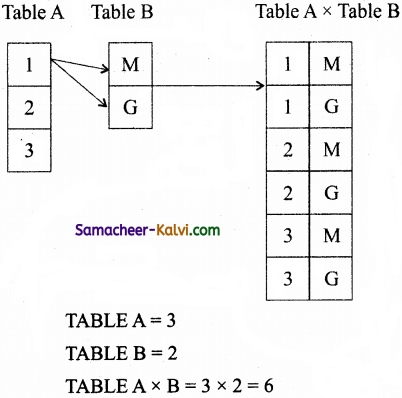Question 30.
Explain Object Model with example.
(i) Object model stores the data in the form of objects, attributes and methods, classes and inheritance.
(ii) It provides a clear modular structure it is easy to maintain and modify the existing code.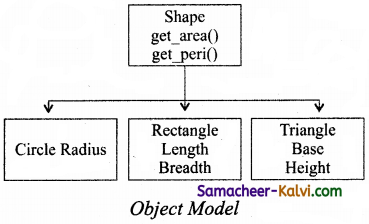(iii) Circle has the attribute radius.
(iv) Rectangle has the attribute length and breadth.
(v) Triangle has the attributes base and height.
(vi) The objects circle, Rectangle and Triangle inherit from the object shape.Question 31.
Write a note on different types of DBMS users.

1. Different Types of database users in DBMS, this differentiation is made according to the interaction of users to the database.
2. As its name shows, application programmers are the one who writes application programs that uses the database.
3. They interact with DBMS through DML (Data manipulation language) calls.
4. Different types of database users are Application programmers. End users, casual users, DBA, system analyst etc.,

Question 32.
Explain the different types of data model.
The different types of data model are
(i) Hierarchical model
(ii) Relational model
(iii) Network Database model
(iv) Entity Relationship model
(v) Object model

(i) Hierarchical model:
This model represents a one-to-many relationship i.e., parent-child relationship.
This model is mainly used in IBM main frame computers.

(ii) Relational model:
The basic structure of data in relational model is tables. All the information’s related to a particular type is stored in rows of that table.Hence tables are also known as relations in a relational model.

(iii) Network Database model:
In a Network model, a child may have many parent nodes. It represents the data in many-to-many relationships.
This model is easier and faster to access the data.

(iv) Entity Relationship model (ER mode):
In this model, relationship are created by dividing the object into entity and its characteristics into attributes.
Eg: Rectangle represents the entities, Doctor and patient.

(v) Object model: This model stores the data in the form of objects, attributes and methods, classes and inheritance.
This model handles more complex applications, such as Geographic information system (GIS), scientific experiments, engineering design and manufacturing.
Eg: Rectangle has the attributes length and breadth.Question 33.
Explain the different types of relationship mapping.
The different types of relationship map used in a database are:
(i) One – to – One Relationship
(ii) One – to – Many Relationship
(iii) Many – to – One Relationship
(iv) Many – to – Many Relationship

(i) One – to – One Relationship:
One entity is related with only one other entity.
One row in a table is linked with only one row in another table and vice versa.
Eg: A student can have only one exam number.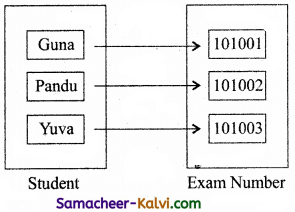(ii) One – to – Many Relationship:
One entity is related to many other entities.
Eg: Department has many staff members.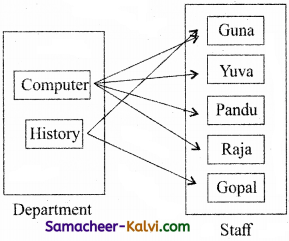(iii) Many – to – One Relationship:
Many entities can be related with only one in the other entity.
Eg: Many staff members working in one department.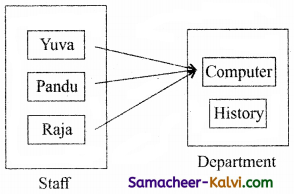(iv) Many – to – Many Relationship:
A many – to – many relationship occurs when multiple records in a table are associated with multiple records in another table.
Eg: Many books in a Library are issued to many students.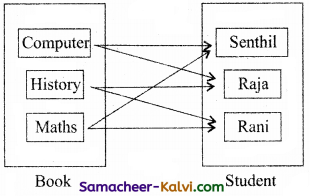Question 34.
Differentiate DBMS and RDBMS.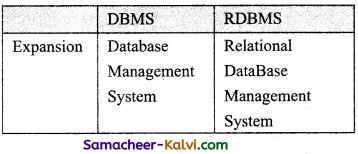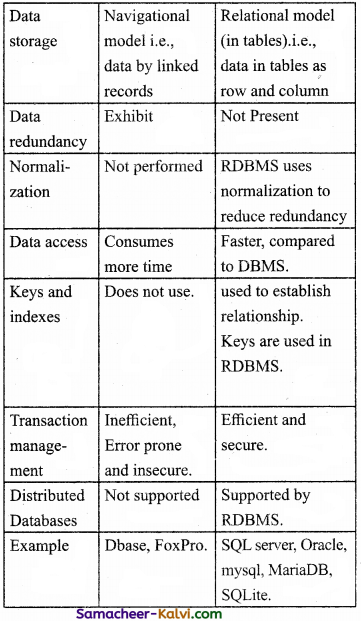Question 35.
Explain the different operators in Relational algebra with suitable examples.
Relational Algebra is a procedural query language used to query the database tables using SQL.
Relational Algebra is divided into various groups.
Unary Relational operations
SELECT (symbol :σ)
PROJECT (symbol :Π)

SELECT (σ):
The SELECT operation is used for selecting a subset with tuples according to a given condition.
The general form ac (R) with a relation R and a condition C on the attributes of R.
Eg:

 Student No. Name Class 1001 Raju 12 1002 Vasanth 11 1003 Murugan 12

σ = 12

 Student No. Name Class 1001 Raju 12 1003 Murugan 12

PROJECT(Π):
The projection eliminates all attributes of the input relation but those mentioned in the projection list.
Eg:
ΠClass (student)
Class
12
11
12
Relational Algebra operations from set theory
• UNION (∪)
• INTERSECTION (∩)
• DIFFERENCE (-)
• CARTESIAN PRODUCT (×)

UNOIN:
Set A union Set B would be expressed as A∪B
Eg: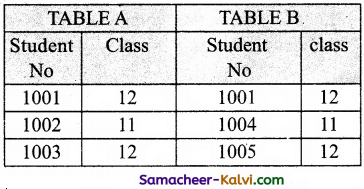Result: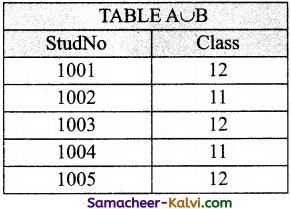SET DIFFERENCE:
A-B is a relation which includes all tuples that are in A but not in B.
Eg: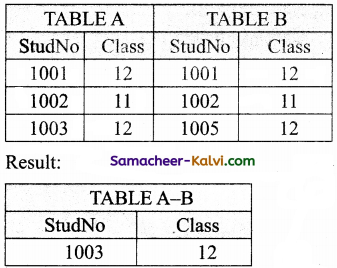INTERSECTION:
A relation consisting of a set of all tuple that are in both in A and B.
Eg: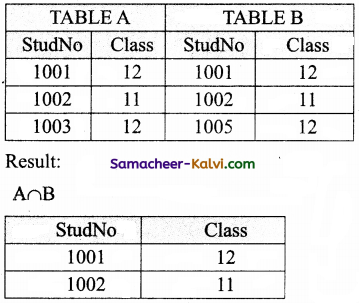CARTESIAN PRODUCT:
A×B means A times B, where the relation A and B have different attributes.
Eg: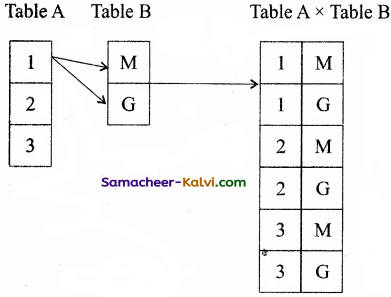Table A = 3
Table B = 2
Table A × B = 6Question 36.
Explain the characteristics of DBMS.
(i) Data stored into Tables:
Data is never directly stored into the database. Data is stored into tables, created inside the database. DBMS also allows to have relationship between tables which makes the data more meaningful and connected.

(ii) Reduced Redundancy:
In the modem world hard drives are very cheap, but earlier when hard drives were too expensive, unnecessary repetition of data in database was a big problem But DBMS follows Normalisation which divides the data in such a way that repetition is minimum.

(iii) Data Consistency:
On live data, it is being continuously updated and added, maintaining the consistency of data can become a challenge.But DBMS handles it by itself.

(iv) Support Multiple user and Concurrent Access:
DBMS allows multiple users to work on it (update, insert, delete data) at the same time and still manages to maintain the data consistency.

(v) Query Language:
DBMS provides users with a simple query language, using which data can be easily fetched, inserted, deleted and updated in a database.

(vi) Security:
The DBMS’also takes care of the security of data, protecting the data from unauthorized access. In a typical DBMS, we can create user accounts with different access permissions, using which we can easily secure our data by restricting user access.

(vii) DBMS Supports Transactions:
It allows us to better handle and manage data integrity in real world applications where multi-threading is extensively used.Choose the best answer:

Question 1.
_________ is an organized collection of data.
(a) Database
(b) Record
(c) File
(d) Data
(a) Database

Question 2.
Which software that allows us to create, define and manipulate database.
(a) DBMS
(b) OS
(d) word
(a) DBMS

Question 3.
Which is maintaining the consistency of data can become a challenge when it live data is being continuously updated and added?
(a) Reduced Redundancy
(b) Data consistency
(c) Concurrent Access
(d) DBMS Supports
(b) Data consistencyQuestion 4.
Which is called the entire collection of related data in one Table?
(a) Record
(b) File
(c) Field
(d) database
(b) File

Question 5.
Each row in a Table represents a:
(a) Table
(b) File
(c) record
(d) field
(c) record

Question 6.
Each Table column represents a:
(a) Table
(b) File
(c) record
(d) field
(d) fieldQuestion 7.
A Table is known as:
(a) Relation
(b) Tuple
(c) Attribute
(d) Model
(a) Relation

Question 8.
A Row is known as:
(a) Relation
(b) Tuple
(c) Attribute
(d) Model
(b) Tuple

Question 9.
A column is known as:
(a) Relation
(b) Tuple
(c) Attribute
(d) Model
(c) AttributeQuestion 10.
Who was developed Hierarchical model system?
(a) IBM
(b) WIPRO
(c) HCL
(d) MICRO SOFT
(a) IBM

Question 11.
Which model is easier and faster to access the data?
(a) ER Model
(b) Network model
(c) Relational model
(d) Hierarchical model
(b) Network model

Question 12.
Who manages the complete database management system?
(a) Database Administrator (DBA)
(b) Programmer
(c) System model
(d) Operation Engineer
(a) Database Administrator (DBA)Question 13.
Match the following:

 (i) Hard ware (A) Program (ii) Soft ware (B) Backups (iii) Data (C) Component (iv) Method (D) Fact

(a) (i) – C, (ii) – A, (iii) – D, (iv) – B
(b) (i) – C, (ii) – D, (iii) – B, (iv) – A
(c) (i) – D, (ii) – B, (iii) – A, (iv) – C
(d) (i) – D, (ii) – C, (iii) – A, (iv) – B’
(a) (i) – C, (ii) – A, (iii) – D, (iv) – B

Question 14.
Match the following:

 (i) Table (A) Tuple (ii) Row (B) Relation (iii) Column (C) Table (iv) File (D) Attribute

(a) (i) – B, (ii) – C, (iii) – D, (iv) – A
(b) (i) – B, (ii) – A, (iii) – D, (iv) – C
(c) (i) – C, (ii) – B, (iii) – D, (iv) – A
(d) (i) – C, (ii) – A, (iii) – D, (iv) – B
(b) (i) – B, (ii) – A, (iii) – D, (iv) – C

Question 15.
Match the following:

 (i) Hierarchical (A) Relation Key (ii) Relational (B) Many – to – many (iii) Network (C) Modular structure (iv) Object (D) One – to – many

(a) (i) – D, (ii) – A, (iii) – B, (iv) – C
(b) (i) – D, (ii) – B, (iii) – C, (iv) – A
(c) (i) – B, (ii) – C, (iii) – A, (iv) – D
(d) (i) – B, (ii) – D, (iii) – A, (iv) – C
(a) (i) – D, (ii) – A, (iii) – B, (iv) – CQuestion 16.
Match the following:

 (i) Hierarchical (A) Relation Key (ii) Relational (B) Many – to – many (iii) Network (C) Modular structure (iv) Object (D) One – to – many

(a) (i) – B, (ii) – D, (iii) – A, (iv) – C
(b) (i) – B, (ii) – C, (iii) – A, (iv) – D
(c) (i) – C, (ii) – B, (iii) – D, (iv) – A
(d) (i) – C, (ii) – A, ( iii) – D, (iv) – B
(d) (i) – C, (ii) – A, ( iii) – D, (iv) – B

Question 17.
Match the following:

 (i) SELECT (A) π (ii) PROJECT (B) × (iii) UNION (C) σ (iv) CARTESIAN PRODUCT (D) ∪

(a) (i) – B, (ii) – C, (iii) – D, (iv) – A
(b) (i) – B, (ii) – D, (iii) – A, (iv) – C
(c) (i) – D, (ii) – C, (iii) – A, (iv) – B
(d) (i) – D, (ii) – A, (iii) – B, (iv) – C
(a) (i) – B, (ii) – C, (iii) – D, (iv) – A

Question 18.
Choose the incorrect pair:

 (a) data fact (b) database C++ (c) RDBMS MySQL (d) DBMS FoxPro

(b)Question 19.
Choose the incorrect pair:

 Column I Column II (a) Table Tuple (b) File Table (c) Column Attribute (d) Row Record

(a)

Question 20.
Choose the incorrect pair:
(a) IBM – Hierarchical
(b) Network – Many
(c) ER-Model – EF codd
(d) GIS – Object model
(c) ER-Model – EF codd

Question 21.
Choose the correct pair:
(a) DBA – Database Agent
(b) End user – Programmer
(c) Chen – ER model
(d) SQuestion lite – Compiler
(c) Chen – ER modelQuestion 22.
Choose the correct pair:
(a) DBMS – Multiple user
(b) Duplication – Segregation
(c) Redundancy – Program
(d) Query – Application
(a) DBMS – Multiple user

Question 23.
Choose the incorrect statement.
(a) A database is an organized collection of data.
(b) The term database is also used to refer to any of the DBMS.
(c) DBMS allows only single user to work on and access it.
(d) Dot is never directly stored into the database.
(c) DBMS allows only single user to work on and access it.

Question 24.
Choose the incorrect statement.
(a) The data are fact stored in a computer.
(b) A day may contain any number and special character.
(c) Information is formatted data.
(d) Database also maintain data consistency in case multiple users.
(b) A day may contain any number and special character.Question 25.
Choose the incorrect statement.
(a) The computer, hard disk and any other physical component involved in storage of data.
(b) The main component is software that control I/O devices storage and everything.
(c) An example of DBMS is C++
(d) Data is that resource for which DBMS is designed.
(c) An example of DBMS is C++

Question 26.
Choose the correct statement.
(a) Each row in a table represents a record.
(b) A row is known as a Relation.
(c) A Table is known as a Tuple.
(d) A column is known as a file.
(a) Each row in a table represents a record.

Question 27.
Choose the correct statement.
(a) Hierarchical model was developed by Google.
(b) In Hierarchical model, data is represented as a simple tree like structure form.
(c) Hierarchical model represents a many – to – many relationship.
(d) Hierarchical model is mainly used single user operational system.
(b) In Hierarchical model, data is represented as a simple tree like structure form.Question 28.
Assertion (A):
A basic structure of data in relational model is tables.
Reason (R):
All the information related to a particular type is stored in rows of that table.
(a) Both A and R are True and A is the correct explanation for R.
(b) Both A and R are True but A is not the correct explanation for R.
(c) A is True but R is False.
(d) A is False but R is True.
(a) Both A and R are True and A is the correct explanation for R.

Question 29.
Assertion (A):
In a Network model, a child may have many parent nodes.
Reason (R):
It represents the data in many -to – one relationship.
(a) Both A and R are True and A is the correct explanation for R.
(b) Both A and R are True but A is not the correct explanation for R.
(c) A is True but R is False.
(d) A is False but R is True.
(c) A is True but R is False.

Question 30.
Assertion (A):
Object model stores the data in the form of objects, attributes and methods, classes and inheritance.
Reason (R):
DBA is the one who manages the complete database management system.
(a) Both A and R are True and A is the correct explanation for R.
(b) Both A and R are True but A is not the correct explanation for R.
(c) A is True but R is False.
(d) A is False but R is True
(b) Both A and R are True but A is not the correct explanation for R.Question 31.
Assertion (A):
In One – to – one Relationship, on entity is related with many other entity.
Reason (R):
One row in a table is linked with many rows.
(a) Both A and R are True and A is the correct explanation for R.
(b) Both A and R are True but A is not the correct explanation for R.
(c) A is True but R is False.
(d) A is False but R is True.
(d) A is False but R is True.

Question 32.
Assertion (A):
Relational Algebra was used for modeling the data stored in relational databases and defining queries on it.
Reason (R):
Relational Algebra is a procedural query language used to query the database tables using SQL.
(a) Both A and R are True and A is the correct explanation for R.
(b) Both A and R are True but A is not the correct explanation for R.
(c) A is True but R is False.
(d) A is False but R is True.
(a) Both A and R are True and A is the correct explanation for R.

Question 33.
Pick the odd one out.
(a) dBase
(b) FoxPro
(c) Clipper
(d) UNIX
(d) UNIXQuestion 34.
Pick the odd one out.
(a) RELATION
(b) MySQL
(c) TUPLE
(d) ATTRIBUTE
(b) MySQL

Question 35.
Pick the odd one out.
(a) Object
(b) Methods
(c) DBA
(d) Classes
(c) DBA

Question 36.
Which of the following is an organized collection of data?
(a) Database
(c) Word processor
(d) Worksheet
(a) DatabaseQuestion 37.
Which of the following characteristics of DBMS is challenged one?
(a) Data redundancy
(b) Data security
(c) Data consistency
(d) Data integrity
(c) Data consistency

Question 38.
How many components are there in DBMS?
(a) 2
(b) 3
(c) 4
(d) 5
(d) 5

Question 39.
How many types of data model are there?
(a) 2
(b) 3
(c) 4
(d) 5
(d) 5Question 40.
What is the basic structure of data in relational model?
(a) Rows
(b) Tables
(c) Columns
(d) Fields
(b) Tables

Question 41.
Which year ER – model was developed?
(a) 1972
(b) 1974
(c) 1976
(d) 1978
(c) 1976

Question 42.
Expand GIS:
(a) Geographic Information System
(b) Global Information System
(c) Global Information Service
(d) Geographic Information Service
(a) Geographic Information SystemQuestion 43.
Expand DBA:
(a) Data Base Analyst
(b) Data Bound Analyst
(c) Data Base Administrators
(d) Data Base Advisor
(c) Data Base Administrators

Question 44.
How many types of relationships used in a database?
(a) 2
(b) 3
(c) 4
(d) 5
(c) 4

Question 45.
Expand SQL:
(a) Structured Query Language
(b) Standard Query Language
(c) Structured Quick Learner
(d) Standard Quick Learner
(a) Structured Query LanguageQuestion 46.
Which of the following data model work with Geographic Information System?
(a) Class
(b) Object
(c) Database
(d) Data
(b) Object

Question 47.
How many types of users are there in DBMS?
(a) 2
(b) 3
(c) 4
(d) 5
(c) 4

Question 48.
Who is care of the security in DBMS?
(a) DBA
(b) System Analyst
(c) Program
(d) Data Analyst
(a) DBAQuestion 49.
Expand RDBMS:
(a) Relational Data Base Management System
(b) Redundancy Data Base Management System
(c) Relational Data Base Multiple System
(d) Relative Data Base Management Service.
(a) Relational Data Base Management System

Question 50.
Which symbol represent unary relational operation for SELECT?
(a) σ
(b) π
(c) +
(d) –
(a) σ

Question 51.
Which symbol represent unary relational opration for PROJECT?
(a) σ
(b) π
(c) +
(d) –
(c) +Question 52.
What is the main purpose of data model?
(a) Idea
(b) Data storage
(c) Data Access
(d) Information
(a) Idea

Question 53.
Which data model is represented as a simple tree like structure form?
(a) Relational
(b) Hierarchical
(c) Entity Relationship
(d) Object
(b) Hierarchical

Question 54.
Which key is an attribute that uniquely identifies a particular tuples?
(a) Relation
(b) Entity
(c) Primary
(d) object
(a) RelationQuestion 55.
Which model represent a child has only one parent?
(a) Relational
(b) Hierarchical
(c) Entity Relationship
(d) object
(b) Hierarchical

Question 56.
Which model represent a child may have many parent?
(a) Relational
(b) Hierarchical
(c) Network
(d) Object
(c) Network

Question 57.
Which model represent Doctor and patient?
(a) Relational
(b) Hierarchical
(c) Entity Relationship
(d) Object
(c) Entity RelationshipQuestion 58.
Which model is easy to maintain and modify the existing code?
(a) Relational
(b) Hierarchical
(c) Entity Relationship
(d) Object
(d) Object

Question 59.
Who is involved in developing and designing the parts of DBMS?
(a) Application Programmer
(b) Software Developer
(c) Computer Operator
(d) (a) or (b)
(d) (a) or (b)

Question 60.
Which type of relationship for a student can have only one exam number?
(a) one-to-one
(b) one-to-many
(c) many-to-one
(d) many-to-many
(a) one-to-oneQuestion 61.
Which type of relationship for a school has many students?
(a) one-to-one
(b) one-to-many
(c) many-to-one
(d) many-to-many
(b) one-to-many

Question 62.
Which type of relationship for the teachers are working in one school
(a) one-to-one
(b) one-to-many
(c) many-to-one
(d) many-to-many
(c) many-to-one

Question 63.
Which type of relationship for students can register many courses?
(a) one-to-one
(b) one-to-many
(c) many-to-one
(d) many-to-many
(d) many-to-manyQuestion 64.
Which operation is used for selAnswer:ecting a subset with tuples according to a given condition?
(a) SELECT
(b) PROJECT
(c) UNION
(d) CARTESIAN PRODUCT
(d) CARTESIAN PRODUCT

Question 65.
Which method eliminates all attributes of the input relation but those mentioned in the projection list?
(a) PROJECT ON
(b) SELECT
(c) UNION 4
(d) CARTESIAN PRODUCT
(a) PROJECT ON

Question 66.
Which method defines a relation that contains a vertical subset of Relation?
(a) PROJECTION
(b) SELECT
(c) UNION
(d) CARTESIAN PRODUCT
(a) PROJECTIONQuestion 67.
Which Relational algebra operation is eliminates duplicates?
(a) DIFFERENCE (-)
(b) UNION (∪)
(c) INTERSECTION (∩)
(d) PRODUCT (×)
(b) UNION (∪)

Question 68.
Which Relational algebra operation that includes all tuples that are in A but not in B?
(a) UNION (∪)
(b) DIFFERENCE (-)
(c) INTERSECTION (∩)
(d) PRODUCT (×)
(b) DIFFERENCE (-)

Question 69.
Which type of operation is helpful to merge column from two relations?
(a) UNION (∪)
(b) DIFFERENCE (-)
(c) INTERSECTION (∩)
(d) PRODUCT (×)
(d) PRODUCT (×)Question 70.
What is the acronym of DBMS?
(a) DataBase Management Symbol
(b) Database Managing System
(c) DataBase Management System
(d) DataBasic Management System
(c) DataBase Management System

Question 71.
A table is known as:
(a) tuple
(b) attribute
(c) relation
(d) identity
(c) relation

Question 72.
Which database model represents parent- child relationship?
(a) Relational
(b) Network
(c) Hierarchical
(d) Object
(c) HierarchicalQuestion 73.
Relational database model was first proposed by:
(a) E F Codd
(b) EE Codd
(c) E F Cadd
(d) E F Codder
(a) E F Codd

Question 74.
What type of relationship does hierarchical model represents?
(a) one-to-one
(b) one-to-many
(c) many-to-one
(d) many-to-many
(b) one-to-many

Question 75.
Who is called Father of Relational Database from the following?
(a) Chris Date
(b) Hugh Darween
(c) Edgar Frank Codd
(d) Edgar Frank Cadd
(c) Edgar Frank CoddQuestion 76.
Which of the following is an RDBMS?
(a) Dbase
(b) Foxpro
(c) Microsoft Access
(d) SQLite
(d) SQLite

Question 77.
What symbol is used for SELECT statement?
(a) σ
(b) Π
(c) X
(d) Ω
(a) σ

Question 78.
A tuple is also known as:
(a) table
(b) row
(c) attribute
(d) field
(b) rowQuestion 79.
Who developed ER model?
(a) Chen
(b) EF Codd
(c) Chend
(d) Chand